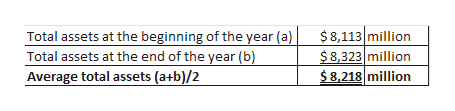# In its 2014 annual report, Campbell Soup Company reports beginning-of-the-year total assets of \$8,113 million, end-of-the-year total assets of \$8,323 million, total sales of \$8,268 million, and net income of \$807 million.  Please answer all parts.   Compute Campbell’s asset turnover. (Round answer to 4 decimal places, e.g. 4.8726.)Asset turnover   times  LINK TO TEXT   Compute Campbell’s profit margin on sales. (Round answer to 4 decimal places, e.g. 4.8726%.)Profit margin on sales   %  LINK TO TEXT   Compute Campbell’s return on assets using (1) asset turnover and profit margin and (2) net income. (Round answers to 2 decimal places, e.g. 4.87%.)    Return on assets (1) Asset turnover and profit margin   %(2) Net income   %

Question
68 views
In its 2014 annual report, Campbell Soup Company reports beginning-of-the-year total assets of \$8,113 million, end-of-the-year total assets of \$8,323 million, total sales of \$8,268 million, and net income of \$807 million.

Compute Campbell’s asset turnover. (Round answer to 4 decimal places, e.g. 4.8726.)

 Asset turnover times

Compute Campbell’s profit margin on sales. (Round answer to 4 decimal places, e.g. 4.8726%.)

 Profit margin on sales %

Compute Campbell’s return on assets using (1) asset turnover and profit margin and (2) net income. (Round answers to 2 decimal places, e.g. 4.87%.)

 Return on assets (1) Asset turnover and profit margin % (2) Net income %
check_circle

star
star
star
star
star
1 Rating
Step 1

Determine average t...help_outlineImage TranscriptioncloseTotal assets at the beginning of the year (a) \$8,113 million \$8,323 million \$8,218 million Total assets at the end of the year (b) Average total assets (a+b)/2 fullscreen

### Want to see the full answer?

See Solution

#### Want to see this answer and more?

Solutions are written by subject experts who are available 24/7. Questions are typically answered within 1 hour.*

See Solution
*Response times may vary by subject and question.
Tagged in